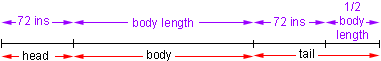I am a student. My name is Bridget. Level of question: secondary. A whale's head is 72 inches long... its tail is as long as its head plus half the length of its body, and its body is half the entire length. How long is the whale? Also, An author published a book every two years. When the seventh one was published, the sum of the years of publication was 13,804. In what year was the author forst published? Would you mind telling me how you discovered the answers please? Hi Bridget, When I read your first question I immediately drew a rough sketch that looked something likeFrom this diagram you can see that if you let 1/2 the body length be x inches Then the total length of the whale is 72 + 2x + 72 + x But you know that the total length is twice the body length, thus 72 + 2x + 72 + x = 2(2x) Solve for x. For the second problem, suppose that y is the year when the first book was published then you can list the years when each of the books was published. The first book was published in y The second book was published in y + 2 The third book was published in y + 4 The fourth book was published in y + 6 . . . Complete the list to include all seven books, set the total of the years to 13,804 and solve for y. Cheers, Penny Go to Math Central